﻿ 基于簇头选举和节点位置优化的WSN分簇路由算法
«上一篇文章快速检索 高级检索

 应用科技2018, Vol. 45Issue (5): 56-61  DOI: 10.11991/yykj.2017100020

### 引用本文LI Xiangfeng, XI Zhihong, ZHENG Anda, et al. WSN clustering routing algorithm based on cluster head election and node location optimization[J]. Applied Science and Technology, 2018, 45(5), 56-61. DOI: 10.11991/yykj.201710002.### 文章历史

1. 哈尔滨工程大学 信息与通信工程学院，黑龙江 哈尔滨 150001;
2. 江西理工大学 信息工程学院，江西 赣州 341000;
3. 哈尔滨工程大学 计算机科学与技术学院，黑龙江 哈尔滨 150001

WSN clustering routing algorithm based on cluster head election and node location optimization
LI Xiangfeng1, XI Zhihong1, ZHENG Anda2, HAN Ning3
1. College of Information and Communication Engineering, Harbin Engineering University, Harbin 150001, China;
2. School of Information Engineering, Jiangxi University of Science and Technology, Ganzhou 341000, China;
3. College of Computer Science and Technology, Harbin Engineering University, Harbin 150001, China
Abstract: Facing the problems of short stable period of cluster routing protocol and short half surviving node period, as well as unstable situation of cluster head election and emergence of zero cluster head, this paper proposes a cluster routing algorithm based on cluster head election and node location optimization to overcome it. Improve the cluster head election process and modify the update strategy of candidate cluster head set to ensure stability of the cluster head election. Add two parameters–the distance between the nodes and the base station and the distance between nodes and cluster heads as the threshold adjustment factors, adjust the probability of the node being selected as cluster head so as to balance the network energy consumption and prolong the stable period and half surviving node period. The simulation results show that the algorithm can guarantee the best number of cluster heads in each round and avoid the appearance of zero cluster head, and effectively prolong the stable period of wireless sensor network (WSN) and the death period of half nodes in terms of network lifetime.
Keywords: wireless sensor network    cluster head election    distance    threshold    stable period    half surviving node    node position    heterogeneous network

1 无线传感器网络模型 1.1 无线传感器结构

$M \times M$ 大小的分层聚类无线传感器网络中，整个WSN被分为若干个簇，每个簇由一个簇头和一定数量的传感器节点组成。假设在整个无线传感器网络内部署N个传感器节点，将传感器节点定义为 $S = \left\{ {{s_1},{s_2}, \cdots ,{s_N}} \right\}$ 。传感器节点监测到的数据通过直接或者间接多跳的方式传输到其所在簇的簇头，簇头收集其成员节点的数据进行分析融合，同样通过直接或者间接多跳的方式将数据传输到汇聚节点。用户可以通过汇聚节点对传感器网络进行配置和管理，发布监测任务以及收集监测数据。典型的WSN结构示意图如图1所示。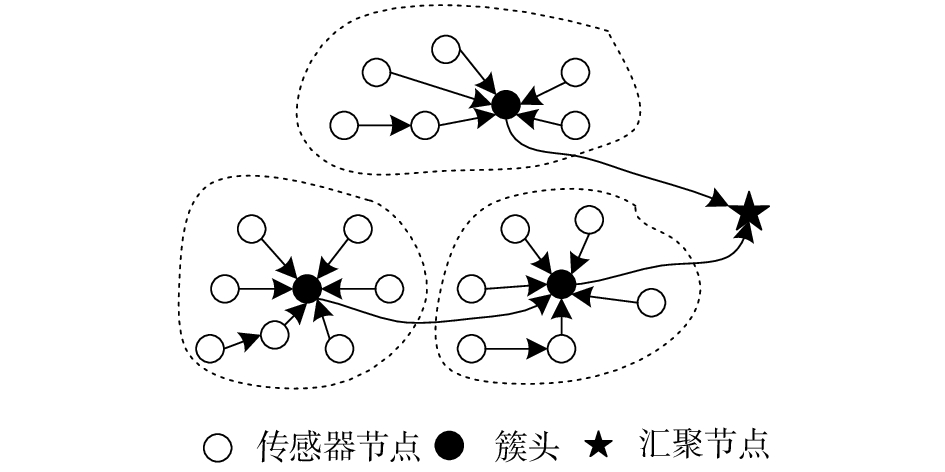Download: 图 1 无线传感器网络示意
1.2 异构网络模型

 $\begin{array}{l}{E_{{\rm{total}}}} = N\left( {1 - m} \right){E_0} + Nm\left( {1 + a} \right){E_0} = \\\;\;\;\;\;\;\;\;\;N{E_0}(1 + am)\end{array}$ (1)

1.3 能量消耗模型

 ${E_{{\rm{Tx}}}}\left( {k,d} \right) = \left\{ {\begin{array}{*{20}{c}} {k{E_{{\rm{elec}}}} + k{E_{{\rm{fs}}}}{d^2},d < {d_0}} \\ {k{E_{{\rm{elec}}}} + k{E_{{\rm{amp}}}}{d^4},d \geqslant {d_0}} \end{array}} \right.$
 ${E_{{\rm{Rx}}}}\left( k \right) = k{E_{{\rm{elec}}}}$
 ${d_0} = \sqrt {\frac{{{E_{{\rm{fs}}}}}}{{{E_{{\rm{amp}}}}}}}$
 ${E_{{\rm{CH}}}} = \left\{ {\begin{array}{*{20}{c}} {k({E_{{\rm{elec}}}} + {E_{{\rm{DA}}}}) + k{E_{{\rm{fs}}}}{d^2},d < {d_0}} \\ {k({E_{{\rm{elec}}}} + {E_{{\rm{DA}}}}) + k{E_{{\rm{amp}}}}{d^4},d \geqslant {d_0}} \end{array}} \right.$

2 基于节点位置优化的分层聚类协议（BCEPOCH）描述 2.1 阈值计算

 {p_i}\left( r \right) = \left\{ {\begin{aligned}& {\displaystyle\frac{{{P_{{\rm{opt}}}}{E_i}(r)}}{{(1 + a \times m)\overline {E} (r)}}{\rm{ }}, \;\; i{\text{是普通节点}}}\\& {\displaystyle\frac{{{P_{{\rm{opt}}}}(1 + a){E_i}(r)}}{{(1 + am)\overline {E}(r) }}{\rm{ }}, \;\; i{\text{是高级节点}}}\end{aligned}} \right.
 ${P_{{\rm{opt}}}} = \sqrt {\frac{1}{{2N{\rm{\pi }}}}} \sqrt {\frac{{{E_{{\rm{fs}}}}}}{{{E_{{\rm{amp}}}}}}} \frac{M}{{d_{{\rm{toSink}}}^2}}$

 $\overline {E}(r) = \frac{{{E_{{\rm{total}}}}(1 - \displaystyle\frac{r}{R})}}{N}$

 ${E_{{\rm{round}}}} = l(2N{E_{{\rm{elec}}}} + N{E_{{\rm{DA}}}} + k{E_{{\rm{amp}}}}d_{{\rm{toSink}}}^4 + N{E_{{\rm{fs}}}}d_{{\rm{toCH}}}^2)$

Heinzelman等定义了节点i的簇头阈值，本文遵循该阈值模型。节点i的阈值计算公式为

 $T\left( i \right) = \left\{ {\begin{split}&{\displaystyle\frac{{{p_i}\left( r \right)}}{{1 - {p_i}\left( r \right)(od (r,1/{p_i}\left( r \right)))}},i{\text{是候选簇头}}}\\&{0,{\text{其他}}}\end{split}} \right.$
2.2 改进簇头选举策略，优化簇头数量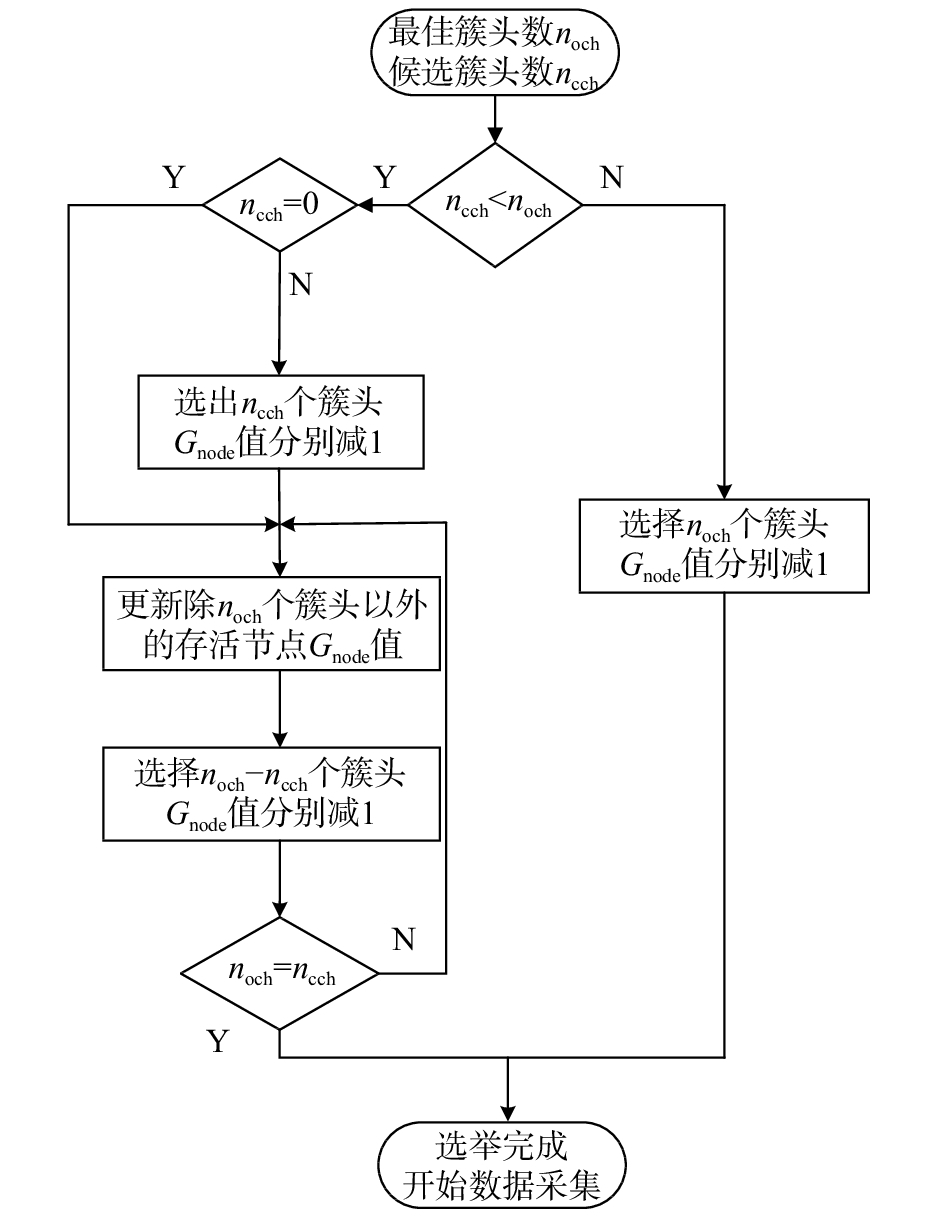Download: 图 2 BCEPOCH簇头选举流程
2.3 节点阈值修正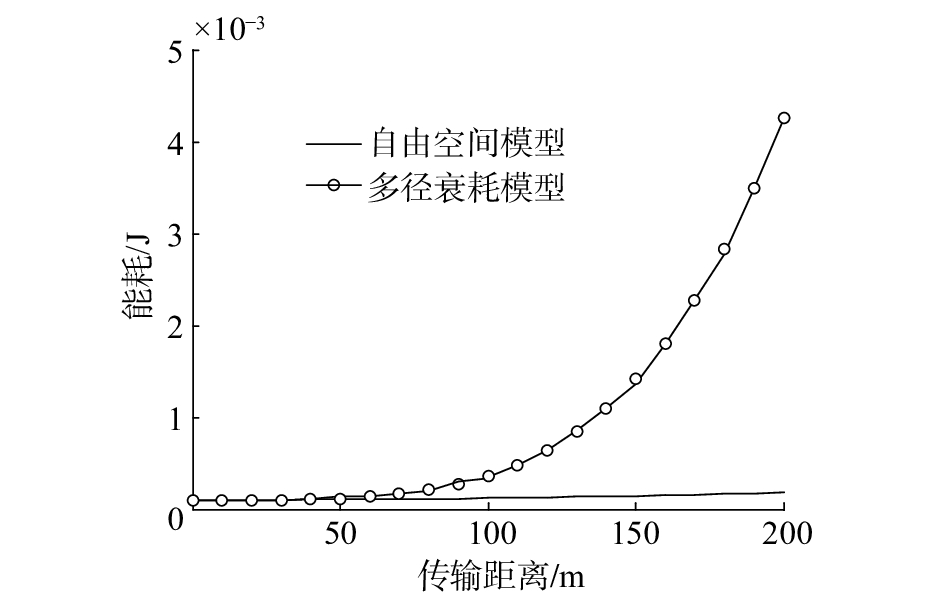Download: 图 3 距离和能耗的关系

 $\varphi \left( {i,j} \right) = {\left( {\frac{{{s_{{\rm{avg\_to\_Sink}}}}}}{{{s_{{\rm{i\_to\_Sink}}}}(i)}}} \right)^\alpha }i \in \left[ {1,N} \right],j = 1$
 $T\left( i \right) = T\left( i \right)\varphi \left( {i,j} \right) = T\left( i \right){\left( {\frac{{{s_{{\rm{avg\_to\_Sink}}}}}}{{{s_{{\rm{i\_to\_Sink}}}}(i)}}} \right)^\alpha }$

 $\begin{array}{l}\varphi \left( {i,j} \right) = {\left( {\displaystyle\frac{{{s_{{\rm{i\_min\_to\_CH}}}}(j)}}{{{s_{{\rm{avg\_min\_to\_CH}}}}}}} \right)^\beta }{\left( {\displaystyle\frac{{{s_{{\rm{avg\_to\_Sink}}}}}}{{{s_{{\rm{i\_to\_Sink}}}}(i)}}} \right)^\alpha }\end{array}$

3 仿真结果及分析表 1 实验参数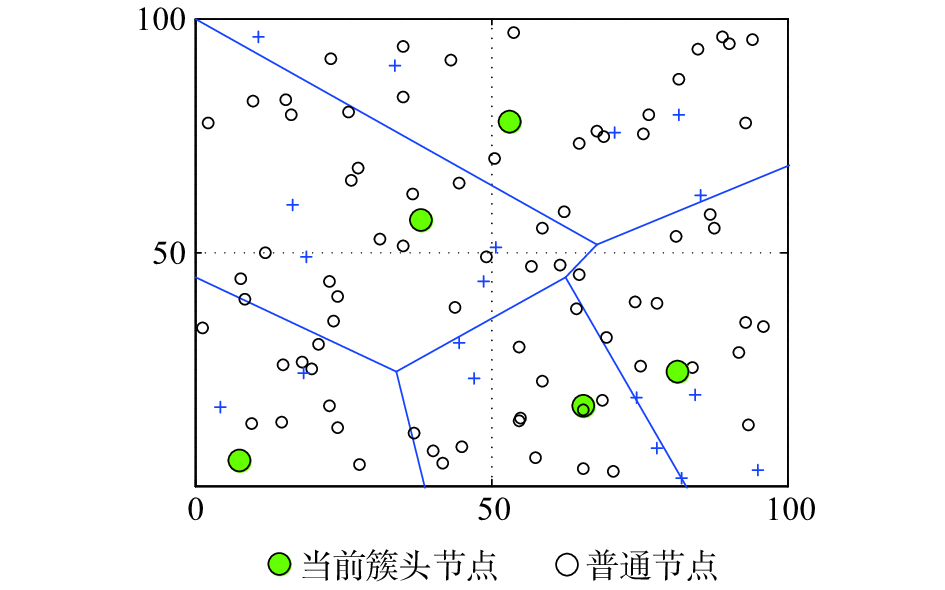Download: 图 4 BCEPOCH运行示意
3.1 簇头选举优化表 2 各算法簇头选举数量分析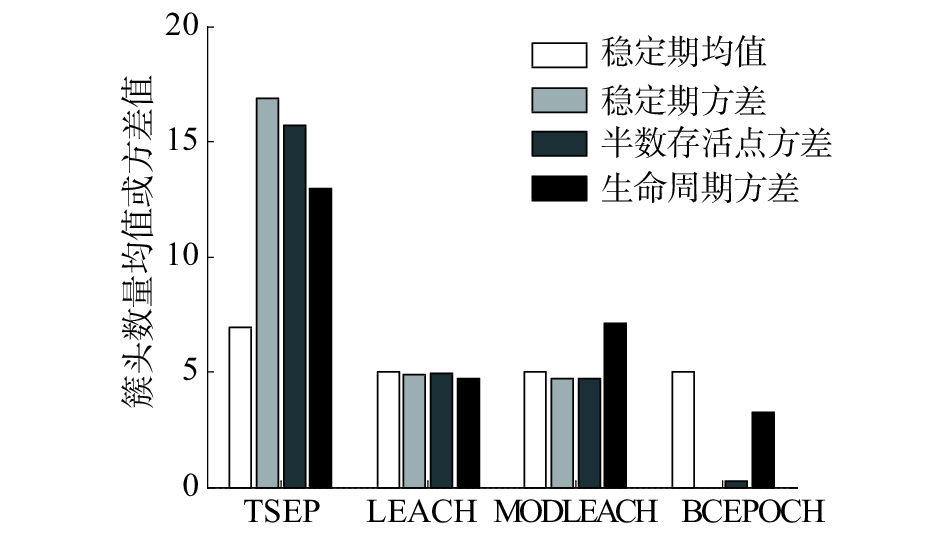Download: 图 5 簇头稳定性分析

3.2 网络有效生命周期和网络寿命表 3 各协议不同阶段的运作轮数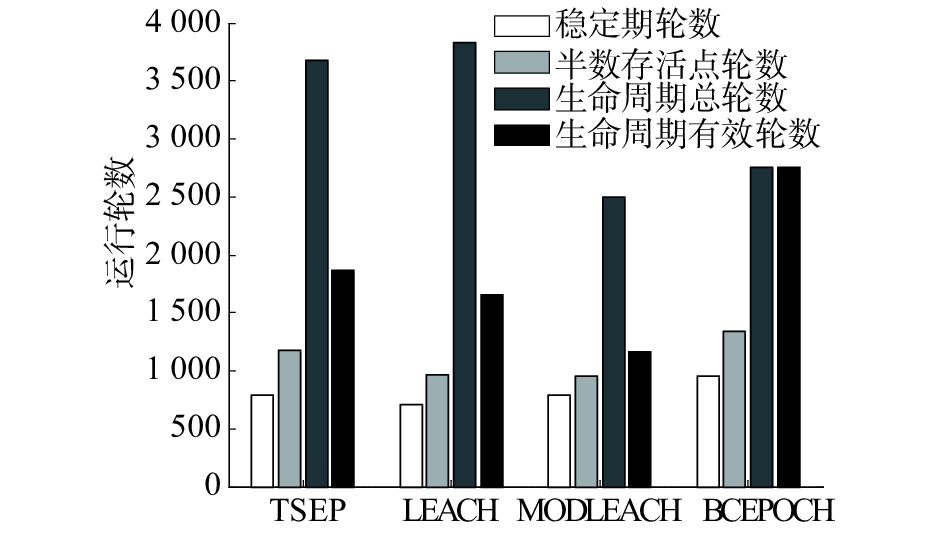Download: 图 6 各时期网络寿命周期对比
4 结论

1）提出的修改簇头选举流程可以有效地保障每轮网络都能选举出合理数量的簇头，避免零簇头的产生，提高簇头选举的稳定性。

2）根据节点与簇头的距离和节点与基站的距离实时修改节点阈值，延长网络的稳定期。

3）本文中仍然存在可以优化的空间，可以看出本文算法在稳定期与生命周期的占比上并不占优势，下一步的研究应当重点关注与提高稳定期的占比上。

  王平, 王恒. 无线传感器网络技术及应用[M]. 北京: 人民邮电出版社, 2016: 3-8. (0)  蔺莉, 张莉华. 无线传感器网络中能量高效的自适应分簇算法[J]. 仪表技术与传感器, 2017(3): 121-126. DOI:10.3969/j.issn.1002-1841.2017.03.031 (0)  LI Qing, ZHU Qingxin, WANG Mingwen. Design of a distributed energy-efficient clustering algorithm for heterogeneous wireless sensor networks[J]. Computer communications, 2006, 29(12): 2230-2237. DOI:10.1016/j.comcom.2006.02.017 (0)  YADAV R, SAXENA S. Improved leach routing protocol with soft computing[C]//Proceedings of the 2nd International Conference on Advances in Computing and Communication Engineering. Dehradun, India: IEEE, 2015: 261-266. (0)  HEINZELMAN W B, CHANDRAKASAN A P, BALAKRISHNAN H. An application-specific protocol architecture for wireless microsensor networks[J]. IEEE transactions on wireless communications, 2002, 1(4): 660-670. DOI:10.1109/TWC.2002.804190 (0)  MAO Lin, ZHANG Ying. An energy-efficient LEACH algorithm for wireless sensor networks[C]//Proceedings of the 36th Chinese Control Conference. Dalian, China: IEEE, 2017: 9005-9009. (0)  李一泓. 基于能量异构无线传感器网络的路由算法研究[D]. 南昌: 南昌大学, 2013. (0)  严英鹏. 基于混合聚类算法的无线传感器网络LEACH协议改进研究[D]. 广州: 华南农业大学, 2016. (0)  LIU Jingjing, HU Yanjun. A balanced and energy-efficient clustering algorithm for heterogeneous wireless sensor networks[C]//Proceedings of the 6th International Conference on Wireless Communications and Signal Processing. Hefei, China: IEEE, 2014: 1-6. (0)  GAO Ying, WKRAM C H, DUAN Jiajie, et al. A novel energy-aware distributed clustering algorithm for heterogeneous wireless sensor networks in the mobile environment[J]. Sensors, 2015, 15(12): 31108-31124. DOI:10.3390/s151229836 (0)  KASHAF A, JAVAID N, KHAN Z A, et al. TSEP: threshold-sensitive stable election protocol for WSNs[C]//Proceedings of the 10th International Conference on Frontiers of Information Technology. Islamabad, India: IEEE, 2012: 164-168. (0)  JAVAID N, QURESHI T N, KHAN A H, et al. EDDEEC: enhanced developed distributed energy-efficient clustering for heterogeneous wireless sensor networks[J]. Procedia computer science, 2013, 19: 914-919. DOI:10.1016/j.procs.2013.06.125 (0)  TIWARI T, ROY N R. Modified DEEC: a varying power level based clustering technique for WSNs[C]//International Conference on Computer and Computational Sciences. Noida, India: IEEE, 2015: 170-176. (0)